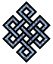#fail2ban bad ip database: ip 93.39.196.245

### | ip database | live view | stats | report | help | api key:

 ip: 93.39.196.245 hostname: 93-39-196-245.ip77.fastwebnet.it country:[IT] Italy first reported: 24.09.2018 04:17.09 GMT+0100 last reported: 03.01.2019 04:21.42 GMT+0100 time period: 101d 01h 04m 33s total reports: 5 reported by: 2 host(s) filter(s): sshd (3) ssh (2) tor exit node no badips.com db Lookup## port scan of '93.39.196.245':

[-hide]
```# Nmap 6.40 scan initiated Mon Sep 24 04:18:02 2018 as: /usr/bin/nmap -sU -sS -O 93.39.196.245
Nmap scan report for 93-39-196-245.ip77.fastwebnet.it (93.39.196.245)
Host is up (0.032s latency).
Not shown: 1000 open|filtered ports, 998 filtered ports
PORT   STATE  SERVICE
22/tcp open   ssh
80/tcp closed http
No OS matches for host (If you know what OS is running on it, see http://nmap.org/submit/ ).
TCP/IP fingerprint:
OS:SCAN(V=6.40%E=4%D=9/24%OT=22%CT=80%CU=%PV=N%G=Y%TM=5BA84975%P=x86_64-pc-
OS:linux-gnu)SEQ(SP=100%GCD=1%ISR=107%TI=Z%CI=RI%TS=U)OPS(O1=M229C%O2=M229C
OS:%O3=M229C%O4=M229C%O5=M229C%O6=M229C)WIN(W1=67D4%W2=67D4%W3=67D4%W4=67D4
OS:%W5=67D4%W6=67D4)ECN(R=Y%DF=Y%TG=40%W=67D4%O=M229C%CC=Y%Q=)T1(R=Y%DF=Y%T
OS:G=40%S=O%A=S+%F=AS%RD=0%Q=)T2(R=Y%DF=Y%TG=40%W=0%S=Z%A=S%F=AR%O=%RD=0%Q=
OS:)T3(R=Y%DF=Y%TG=40%W=0%S=Z%A=O%F=AR%O=%RD=0%Q=)T4(R=N)T5(R=Y%DF=Y%TG=40%
OS:W=0%S=Z%A=S+%F=AR%O=%RD=0%Q=)T6(R=N)T7(R=Y%DF=Y%TG=40%W=0%S=Z%A=S+%F=AR%
OS:O=%RD=0%Q=)U1(R=N)IE(R=Y%DFI=N%TG=40%CD=S)

OS detection performed. Please report any incorrect results at http://nmap.org/submit/ .
# Nmap done at Mon Sep 24 04:18:29 2018 -- 1 IP address (1 host up) scanned in 28.19 seconds
```
```Σ = 31 | Δt = 0.0040619373321533s
```